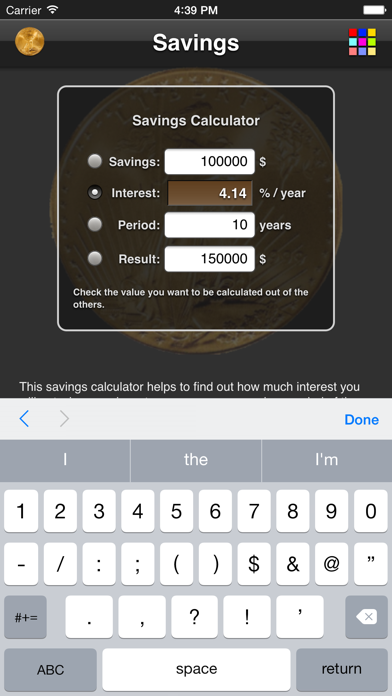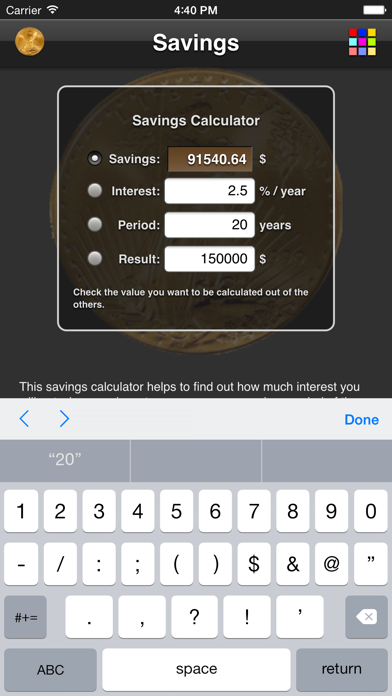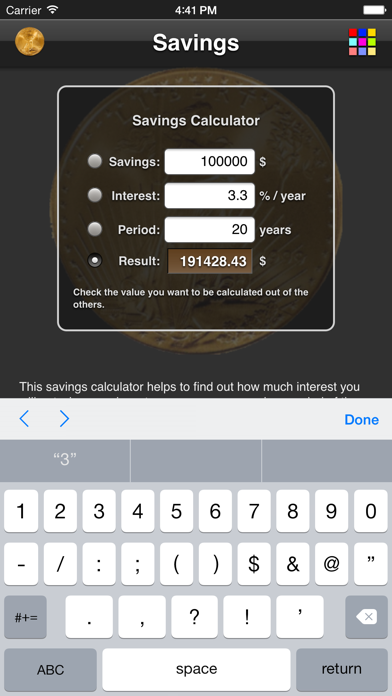# Savings Calculator

## iOS UniversalFinance

\$0.99

This savings calculator helps to find out how much interest you will get when you invest your money over a given period of time with a fixed interest rate. It calculates the compound interest which means it assumes you reinvest the interest after each year (or just leave it in the bank account). So you will profit from the interest you earn on interest you already got.

You can calculate any value in the interest equations, so that you can find out how much interest rate or how many years are necessary to reach a certain goal. As well you can find out how much money you need to start with. This is perfect for doing "what-if" analysis.

Show More...

## What's New

- support for iPhone X+

••••